| Cart Total:

# Gage Repeatability and Reproducibility

Quality Glossary Definition: Gage repeatability and reproducibility (GR&R)

Gage repeatability and reproducibility (GR&R) is defined as the process used to evaluate a gauging instrument’s accuracy by ensuring its measurements are repeatable and reproducible. The process includes taking a series of measurements to certify that the output is the same value as the input, and that the same measurements are obtained under the same operating conditions over a set duration.

## Gage Repeatability

Factors that affect measurement system variation can be studied using the GR&R technique. When using the GR&R system, measurement system variation can be characterized by location (stability, bias, linearity) and width or spread (repeatability and reproducibility).

The GR&R technique can be applied in most manufacturing-related measurement systems. It may be used as:

• A criterion for judging new measuring equipment
• A comparison among measuring devices
• A means for improving performance of measuring instruments
• A comparison for measuring equipment before and after repair
• A required component for calculating process variation and the acceptability level for a production process
• A measure of the need for training in how to use measuring instruments

## Estimating Total Measurement Variation

The "repeatability" aspect of the GR&R technique is defined as the variation in measurement obtained:

• With one measuring instrument
• When used several times by the same operator
• When measuring an identical characteristic on the same part

The standard deviation for repeatability (σe) is estimated using the formula displayed below, where R is the average range of repeated measurements: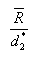The "reproducibility" aspect of the GR&R technique is the variation in the average of measurements made by different operators:

• Who are using the same measuring instrument
• When measuring the identical characteristic on the same part

Operator variation, or reproducibility, is estimated by determining the overall average for each appraiser and then finding the range (R0) by subtracting the smallest operator average from the largest.

The standard deviation for reproducibility (σ0) is estimated by: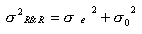The measurement system variation (R&R) or gage R&R is represented by σR&R.

### Part-to-Part Variations in Measurement

Part-to-part variation also makes a contribution to the total variation in a measurement and can be determined from the measurement system data or an independent process capability study.

• If the measurement system study is used, the part standard deviation σp (PV) is estimated by Rp /d2*. Rp can be estimated as the average range of part measurements.

### Total Measurement Variation

Total variation (TV or σTV) for the study is calculated by summing the square of both the repeatability and reproducibility (R&R) variation and the part-to-part variation PV, and taking the square root, as follows: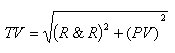The contribution of the equipment variation (EV) is calculated as 100(EV/TV). The contribution of other factors to the total variation TV can be similarly calculated, as follows: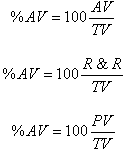## The Three Types of GR&R Studies

There are three GR&R studies—crossed, nested, and expanded—each with a different objective. Choosing which GR&R study to perform depends on how much data are available and whether the measurement test is destructive.

### Crossed GR&R Study

In this study, the same parts are measured multiple times by each operator (Figure 1). It’s used to determine how much process variation is due to measurement system variation.

Crossed GR&R is used in nondestructive scenarios—when parts are not destroyed during measurement and can be measured twice. For example, when measuring the length of a part, the part is not changed during the measurement.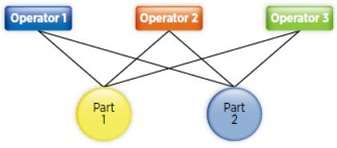Figure 1: Crossed Gage Repeatability and Reproducibility (GR&R) Study

### Nested GR&R Study

This method is used when only one operator measures each part, usually because the test destroys the part (Figure 2). This study is called nested because one or more factors are nested under another factor and not crossed with the other factors.

Nested GR&R is used in destructive testing scenarios. For example, testing the amount of force required to open a bag of potato chips, heat-treating steel tubes, and testing the strength of a rope until it breaks. In these examples, the sample is destroyed during testing, making it impossible to test the sample again.

A critical factor of a nested GR&R study is to identify a batch of material that is so close to the original it reasonably can be assumed the parts in the batch are the same part. The key to being able to run a destructive GR&R study is the assumption that a batch is homogeneous.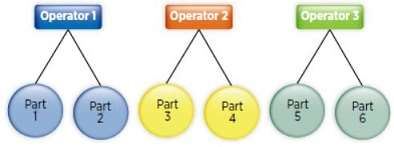Figure 2: Nested Gage Repeatability and Reproducibility (GR&R) Study

### Expanded GR&R Study

Standard GR&R studies (crossed and nested) assess the effects of two factors in the measurement system—typically operator and part (Figure 3). In many cases, the effects of operator and part are not sufficient to provide a complete understanding of the measurement system, so a third variable (typically gage) is added to the standard GR&R study.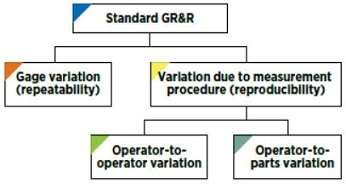Figure 3: Standard Gage Repeatability and Reproducibility (GR&R) Study

When three or more factors are included in the analysis, the study is called expanded GR&R (Figure 4). An expanded GR&R study can be done if one or more of the following conditions exists:

• More than two factors are being assessed. The study analyzes not just parts and operators, but also up to eight additional factors, such as gage, lab, or location.
• There are missing data points. An expanded GR&R study can be performed even with incomplete data and unbalanced studies.
• There are fixed or random factors for greater flexibility.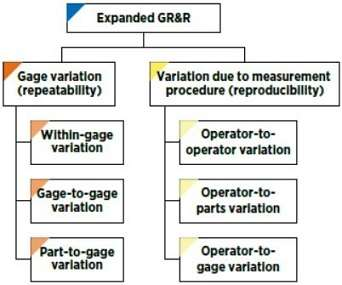Figure 4: Expanded Gage Repeatability and Reproducibility (GR&R) Study

Differences between a standard and expanded GR&R study include:

• The expanded GR&R study allows additional factors to be evaluated.
• Interactions between the additional factors and the operator and part also can be assessed.
• The expanded GR&R study allows analysis of the data—even if there are missing data points.
• The data collection plan commonly is adjusted for the expanded GR&R. Repeating the standard plan for each additional factor is costly, so the number of parts is often reduced. For example, five parts are to be measured by three operators using three randomly selected gages, and each operator will measure each part twice. Thus, the total sample will be 5 × 3 × 3 × 2 = 90. In a standard GR&R study, more parts can be selected, but this is an unacceptably large sample size for expanded GR&R.
• When there isn’t enough data for a standard GR&R study, an expanded GR&R is an ideal tool to comprehensively characterize the measurement system.

## Gage Repeatability and Reproducibility resources

A Study In Measurement (Quality Progress) This article details how gage repeatability and reproducibility studies can determine whether a measurement system is acceptable.

Gauge R&R Studies for Destructive Measurements (Journal of Quality Technology) Alternatives to standard GR&R studies are necessary when objects cannot be measured more than once.

Attribute Gage R&R (Lean & Six Sigma Review) This case study details the measurement phase of a Six Sigma project and shows how attribute GR&R saved one company \$400,000 a year.

Adapted from The Certified Quality Technician Handbook, ASQ Quality Press and "A Study in Measurement," Quality Progress